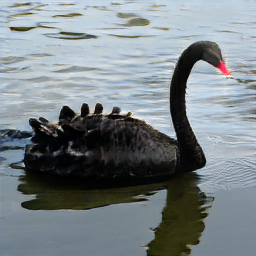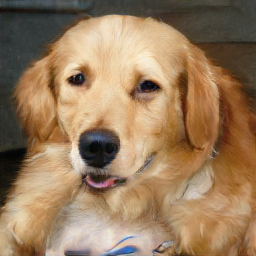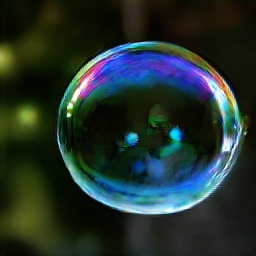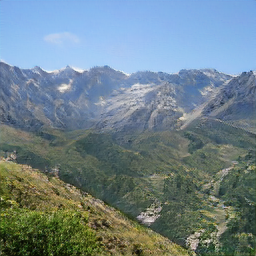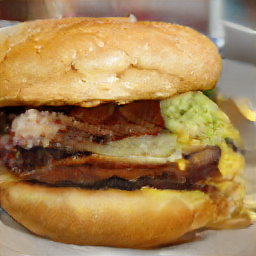# Image Generation with BigGAN from the Model Zoo

Generative Adversarial Networks (GANs) are a branch of deep learning used for generative modeling. They consist of 2 neural networks that act as adversaries, the Generator and the Discriminator. The Generator is assigned to generate fake images that look real, and the Discriminator needs to correctly identify the fake ones.

In this example, you will learn how to use a BigGAN generator to create images, using the generator directly from the ModelZoo.

The source code for this example can be found at BigGAN.java.

## Run Generation

### Introduction

BigGAN is trained on a subset of the ImageNet dataset with 1000 categories. You can see the labels in this file. The training was done such that the input to the model uses the ID of the category, between 0 and 999. For us, the ID is the line number in the file, starting at 0.

Thus, the input to the translator will be an array of category IDs:

``````int[] input = {100, 207, 971, 970, 933};
``````

### Build the project and run

Use the following commands to run the project:

``````cd examples